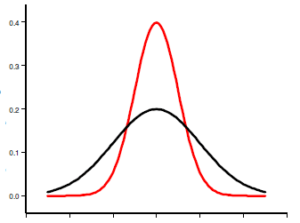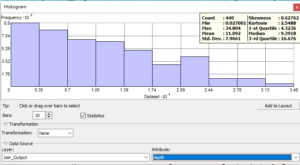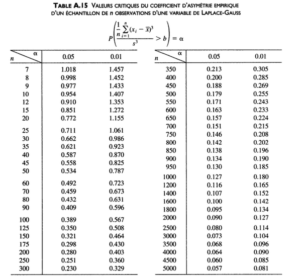# Exploratory Data Analysis for Geostatistics: the Histograms

Following the article Introduction to Exploratory Data Analysis for Geostatistics , we will begin to address each of the tools available for performing the exploratory analysis of spatialized data. We will start with the histograms.

## Histograms

Even if we will use Geostatiscal Analyst tools from ArcGis as a base, you will find similar tools in other GIS software (SAGA tools in QGis …).

The AEDS histogram tool provides a univariate description (one variable) of your data. The tool displays the frequency distribution for the data set of interest and calculates the statistical summary. The primary objective is to validate the fact that the distribution of the values ​​of each variable observes a random phenomenon.

## Frequency distribution

Frequency distribution is a bar graph that indicates the frequency observed by values ​​ in certain ranges or classes. You specify the number of classes of equal width that should be used in the histogram. The relative proportion of data that falls into each class is represented by the height of each bar. For example, the histogram above shows the frequency distribution (10 classes) for a data set.The main characteristics of a distribution can be summarized by some statistical values that describe its distribution, spreading and shape.

## Measures distribution

The values of the distribution provide an idea of ​​where the center and other parts of the distribution are. In terms of our goal, validating a random distribution, these values provide very little. However, they inform us about the data set in order to better understand its characteristics. If we want to make a class symbology, it is very useful to thoroughly understand these values.

The average is the arithmetic mean of the data. The average provides a measure of the center of the distribution.

The median value corresponds to a cumulative proportion of 0.5. If the data is ranked in ascending order, 50% of the values ​​would be below the median and 50% of the values ​​would be above the median. The median provides another measure of the center of the distribution.

The first and third quartiles represent a cumulative proportion of 0.25 and 0.75, respectively. If the data were ranked in ascending order, 25% of the values ​​would be below the first quartile and 25% of the values ​​would be above the third quartile.

If you want a classification, into four classes of equal importance (number of points), you just need to use the first quartile, the median and the third quartile as boundaries. You will have 25% of your data in each class.

The difference in points around the average value is another characteristic of the displayed frequency distribution. The variance of the data is the mean squared deviation ​​from the mean of all the values. The units are the square of the units of the original measures and, because they imply squared differences, the calculated variance is sensitive to abnormally high or low values.
The standard deviation is the square root of the variance. It describes the distribution of data on the average in the same units as the original measurements.

In the previous example, the average value is 0.22705 and the standard deviation is 0.083076. Roughly speaking, this means that 68% of our data will be within the range 0.14 to 0.30.

The larger the standard deviation, the flatter the distribution curve. The smaller the standard deviation, the sharper the curve. The problem on a day-to-day basis is that this applies to each type of data and that there is no point in comparing the standard temperature difference with the standard deviation of the sea ice surface, as the units have no connection.

It’s much simpler to look at the look of the distribution, you’ll see right away if you’re facing a flattened or sharp distribution!## Shape measures

The frequency distribution is also characterized by its shape. And it is here that we have the most important elements to determine whether the distribution of our data follows a normal distribution or not.
The asymmetry coefficient (Skewness) is a measure of the symmetry of a distribution. For symmetric distributions, the asymmetry coefficient is zero. The mean is larger than the median for positive asymmetric distributions, and vice versa for negatively skewed distributions. The figure below shows a positively biased distribution.In the case of a normal distribution, the value of the asymmetry coefficient is 0. But if it is not a perfect match, how should we interpret the result? In our first histogram example the asymmetry coefficient is -0.17. Is it significantly different from 0?

There are many ways to answer this question. Let’s remember here the simplest, without any additional calculations. This is the table found in the book Probability, data analysis and statistics” G.   Saporta (Technip edition) p. 587. This table indicates, for a certain number n of values ​​of the histogram, the values ​​not to be exceeded.Values ​​are given for risks of 1% and 5% for n between 7 to 5000. In our example, considering a sample of 450 observations and an error risk of 5%, the coefficient must be between -0.188 and 0.188 to consider that the distribution is indeed symmetrical.

We are fine in this case.

The flattening coefficient (kurtosis) is based on the height of the edges (or tails) of a distribution and provides a measure of the probability for the distribution to produce outliers, ie values ​​that deviate significantly from the mean .

The kurtosis of a normal distribution is equal to 3. The distributions with relatively thick edges are called   “Leptokurtiques” and have a kurtosis value greater than 3. Distributions with relatively thin edges are called   “Platykurtiques” and have a kurtosis value less than 3. In the figure below, a normal distribution is given in red, and a leptokurtic distribution (thick edges) in blackOn the data set corresponding to the black curve, it will be more difficult to know if the higher or lower values are outliers, ie measurement errors.

In summary: if the kurtosis is less than 3, you will be encouraged to search for outliers, for example using the Voronoi polygons (which we will discuss in a later article), if it is greater than 3, it will be more difficult.

Depending on the tool used, you can calculate another value instead of kurtosis, the excess of kurtosis. It is simply the kurtosis minus 3. Since the value 3 is the central value, the excess of kurtosis makes it possible to immediately recognize the platikurtic curves (negative values ​​of the excess) of the leptokurtiques (positive values).

## Interpretation of histograms

Some kriging methods work best if the data is approximately normally distributed (the bell-shaped curve).
In particular, quartile and probability maps using ordinary, simple and universal kriging assume that the data come from a normal distribution.
As we discussed in the previous article, kriging is also based on the hypothesis of stationarity. This assumption requires, partially, that all data values originate in distributions that bear the same variability. Usually, we observe in nature that as the values ​​increase, their variability increases as well. The transformations of the data source can be used to make your data normally distributed and satisfy the assumption of equal variability for the whole set.
In the Geostatistical Analyst histogram tools, you will find several types of transformations, including the Box Cox (also known as exponential transformation), logarithmic and arc sinus.The simple observation (plus the Skewness value significantly differs from 0 according to the overflow table) indicates that the distribution is not normal.

If we select a Box Cox transformation with a parameter value (exponential function power) of O.55 we haveThe Skewness is now practically 0 (0.0077).

It will be enough to transform the input data with this function so that the geostatistical tools work properly. In Geostatistical Analyst, there is no need to transform the input data. Just indicate the transformation to be done and the data will be transformed automatically before performing the geostatistical calculations, then the results will be transformed with the inverse transformation automatically.

If you wonder how we found the value 0.55, it is not complicated. Each time you change the value of the parameter, the display is recalculated. You immediately see the value of Skewness. By iterations, you will approach the value 0.

In the next article we will discuss another tool of the exploratory analysis of spatialized data: the QQ-plots (quartile-quartile diagrams).